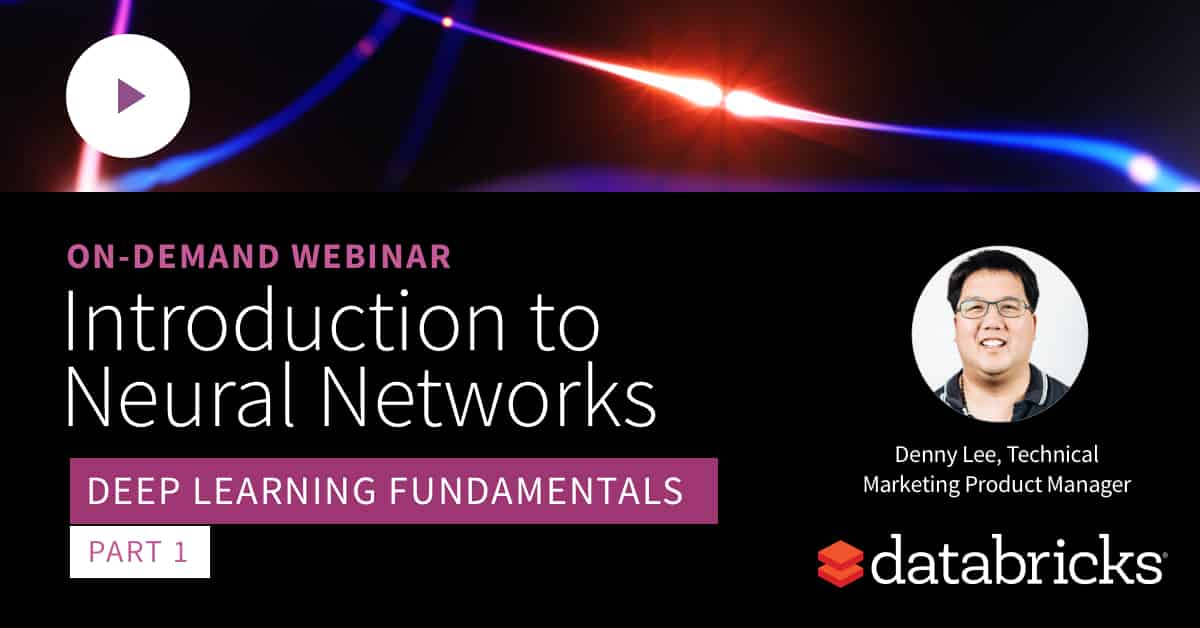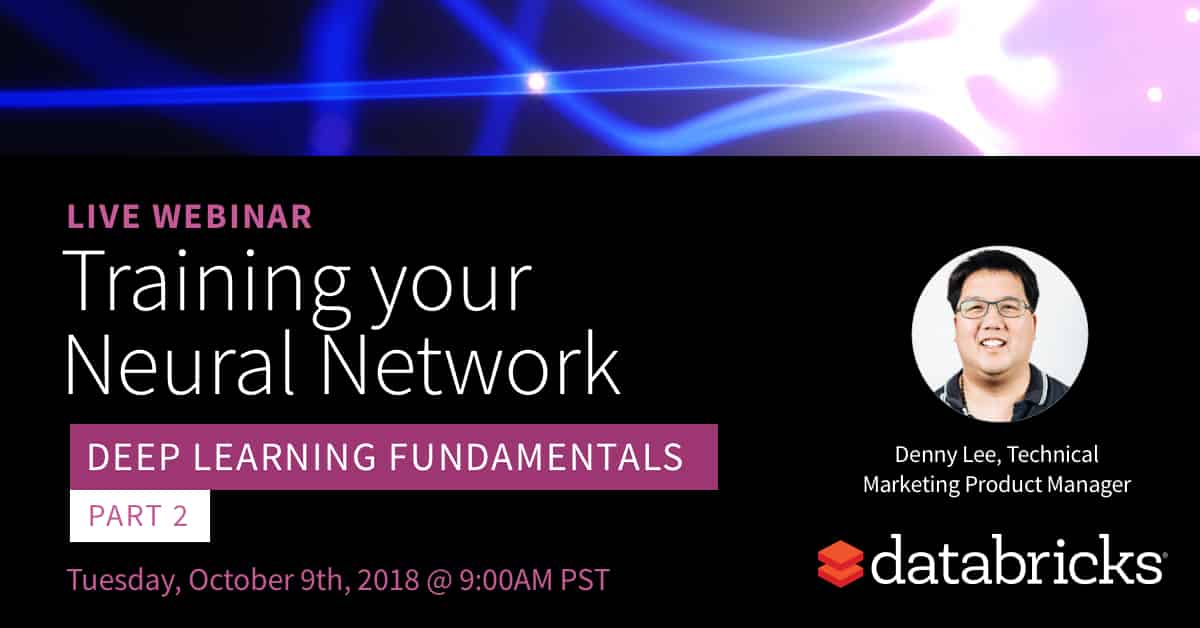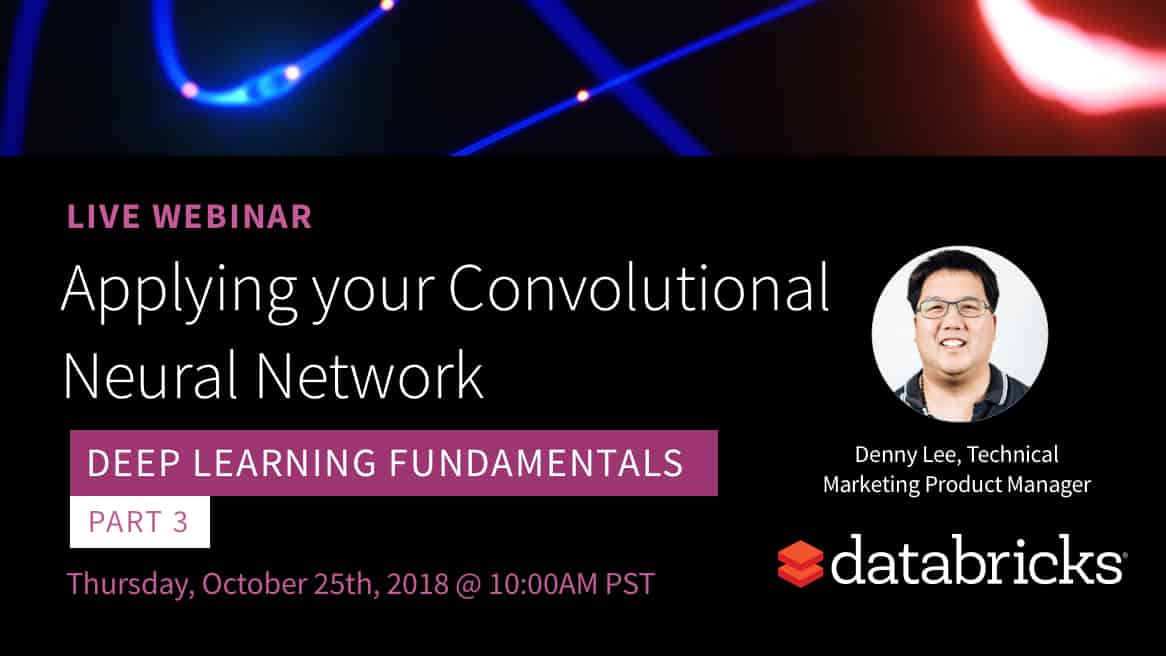# Introduction to Neural Networks: On-Demand Webinar and FAQ Now Available!

Try this notebook in Databricks

On September 27th, we hosted a live webinar—Introduction to Neural Networks—with Denny Lee, Technical Product Marketing Manager at Databricks. This is the first webinar of a free deep learning fundamental series from Databricks.In this webinar, we covered the fundamentals of deep learning to better understand what gives neural networks their expressive power:

• the potential of deep learning and examples of possible applications
• the mathematical concept behind artificial neural networks (ANN)
• the concepts of gradient-descent optimization, backpropagation, and activation functions

We demonstrated some of these concepts using Keras (TensorFlow backend) on Databricks, and here is a link to our notebook to get started today MNIST demo using Keras CNN on Databricks

You can now register to Part 2 and Part 3 where we will continue to explore the power of Neural Networks, and dive more into best-practices to train your neural networks (from preparing your datasets to parameters tuning) as well as architecture examples of Convolutional Neural Networks:If you’d like free access Databricks Unified Analytics Platform and try our notebooks on it, you can access a free trial here.

Toward the end, we held a Q&A, and below are the questions and their answers, grouped by topics.

### Fundamentals

Q: Which activation function is preferred? or which cases suit a particular activation function?

Using ReLU as your activation function is a good starting point as noted in many neural networks samples Keras MNIST,  TensorFlow CIFAR10 Pruning, etc.).  Note that each activation function has its own strengths and weaknesses.  A good quote on activation functions from CS231N summarizes the choice well:

“What neuron type should I use?” Use the ReLU non-linearity, be careful with your learning rates and possibly monitor the fraction of “dead” units in a network. If this concerns you, give Leaky ReLU or Maxout a try. Never use sigmoid. Try tanh, but expect it to work worse than ReLU/Maxout.

Q: On what basis do you select the number of layers and number of neurons in each layer?

In general, the more layers and the number of units in each layer, the greater the capacity of the artificial neural network.  The key concern is you may run the risk over overfitting when your goal is to build a generalized model.

From a practical perspective, a good starting point is:

• The number of input units equals the dimension of features
• The number of output units equals the number of classes (e.g. in the MNIST dataset, there are 10 possible values represents digits (0...9) hence there are 10 output units
• Start with one hidden layer that is 2x the number of input units

A good reference is Andrew Ng’s Coursera Machine Learning course.

Q: What is the ideal training and testing data split size for training deep learning models ?

The split size for deep learning models isn’t that different from general rules of Machine Learning; using an 80/20 split is a good starting point.

### Architecture/Ecosystem

Q: What type of Azure VM should you use to train neural networks (and how much memory)?

A good starting point with would be to utilize clusters with GPU nodes (as of this writing, for Azure this would the N-series VMs) and enough memory to hold a size-able portion of the data.

### Deep Learning on Databricks

Q: Does Databricks provides step by step tutorial which can help to develop some pilots using Artificial Neural Networks (ANN)?

Yes, absolutely, we encourage you to get started with this notebook to explore the potential of ANN. As well, for more examples, refer to the Deep Learning sections of the Databricks Documentation (AWS | Azure).